# 978-0123869449 Chapter 11 Part 3

Document Type
Homework Help
Book Title
Authors
Michael F. Modest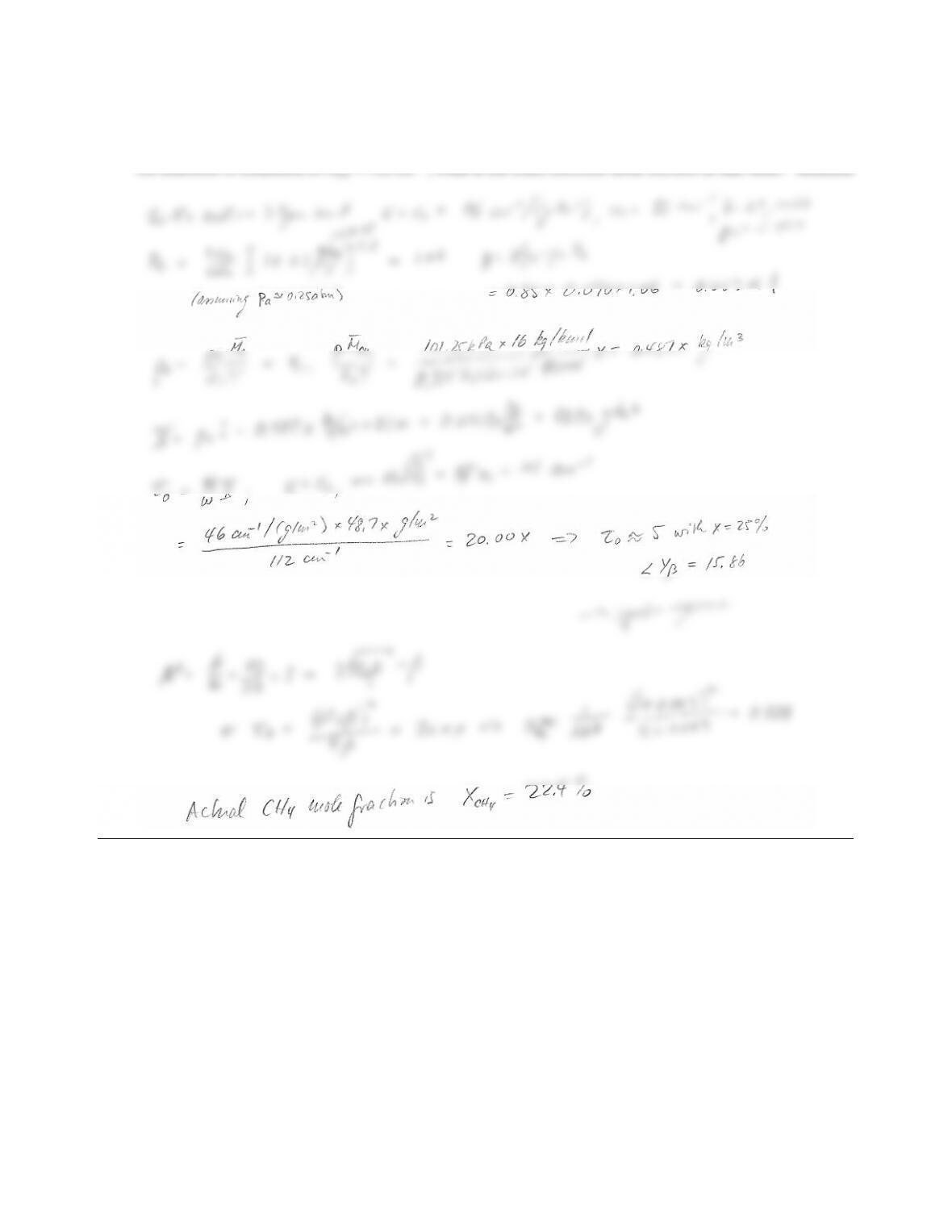11.32 In a combustor the air-fuel ratio is controlled by measuring the total band absorptance of the fuel (methane)
for its 3.3µm band. The mixtures inlet conditions are 1 atm total pressure, temperature is 400 K, combustor
diameter is L=10 cm, and the design mole fraction for methane is 25%. If the total band absorptance across
the diameter is measured as A3.3=112 cm1, what is the exact methane mole fraction at that time? Solution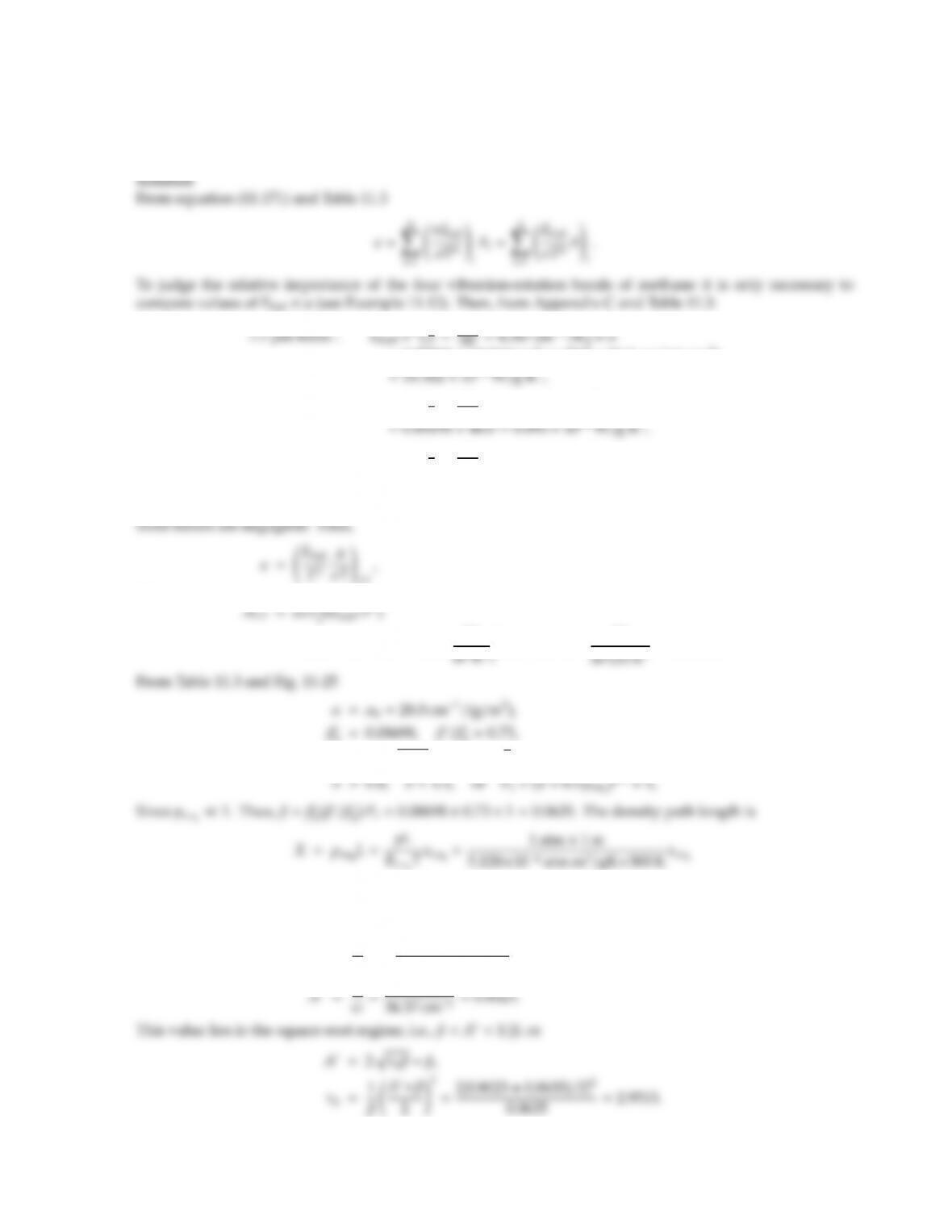CHAPTER 11 325
11.33 A 1 m thick layer of a mixture of nitrogen and methane (CH4) at T =300 K and p=1 atm has a measured total
emissivity of ǫ=0.010. Estimate the partial pressure of the methane (RCH4=5.128 ×106atm m3/g K). It is
known that pCH4p.
=0.58292 ×108W/m2cm1K3×28.0 cm1/(g/m2)
3.3µm band : Ebη0/T3η
T=3020
300 =10.067 cm1/K×α
2.4µm band : Ebη0/T3η
T=4220
300 =14.067 cm1/K×α
=0.00002 ×2.9=0.00006 ×108W/g K3.
It is clear that, due to the spectral positions of the bands, only the 7.7µm needs to be considered; the other
or
=0.010×5.670×108×300 W
0=0.08698, β
00.73,
ω=ω0pT/T0=21.0×3=36.37 cm1,
=650.0xCH4g/m2,
where xCH4is the mole fraction of CH4. Now
τ0=α
ωX=28.0 cm1/(g/m2)
36.37 cm1650.0xg/m2=500.4xCH4,500.4=5.90 ×103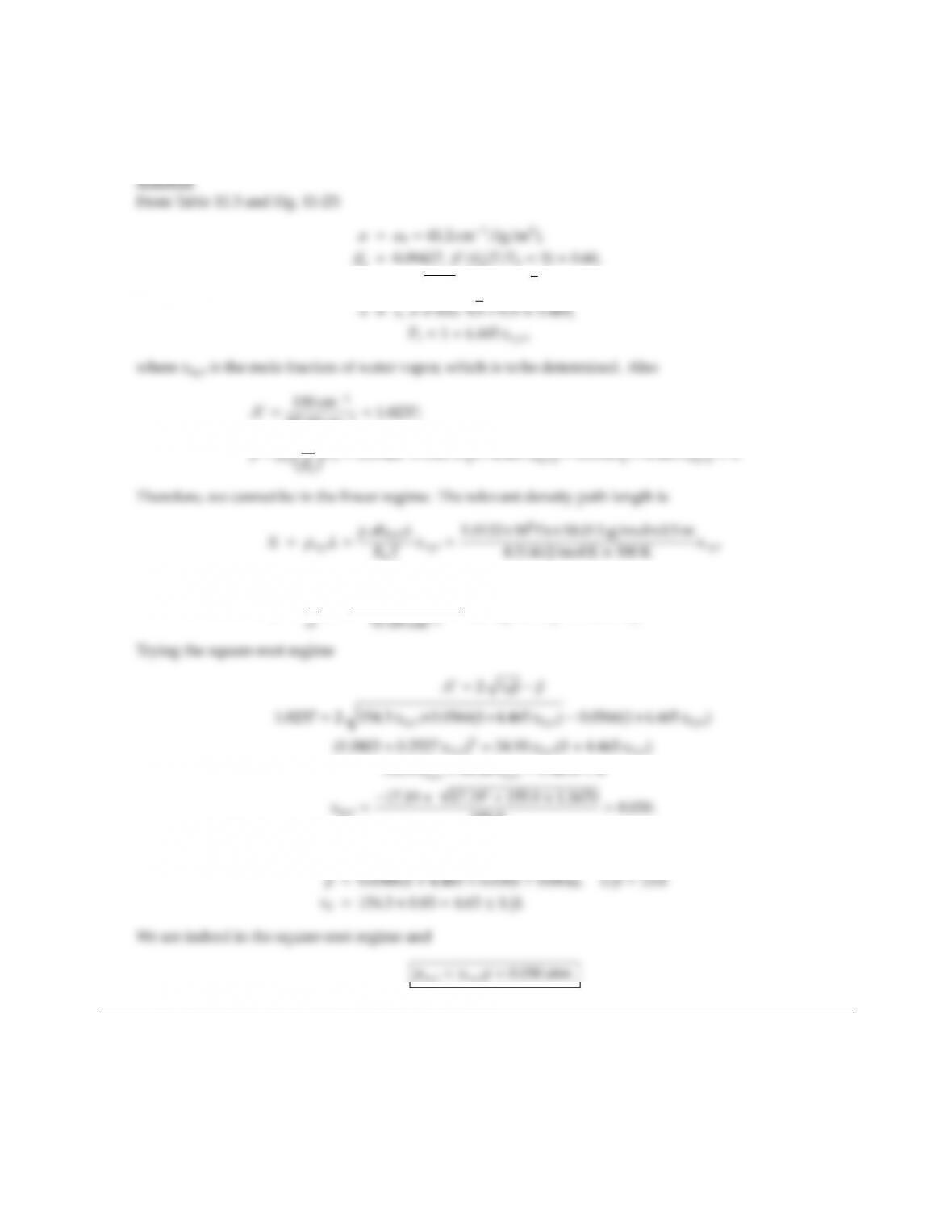CHAPTER 11 327
11.34 A mixture of water vapor and nitrogen at a total pressure of 1 atm and a temperature of 300 K is found to have
a total band absorptance of 100 cm1for the 6.3µm band for a geometric path length of 50 cm. Determine the
partial pressure of the water vapor.
0=0.09427, β
0(T/T0=3) 0.60,
ω=ω0pT/T0=56.4×3=97.69 cm1,
97.69 cm1=1.0237;
β=β
=365.9xH2Og/m2,
τ0=α
155.9=0.030.
Checking,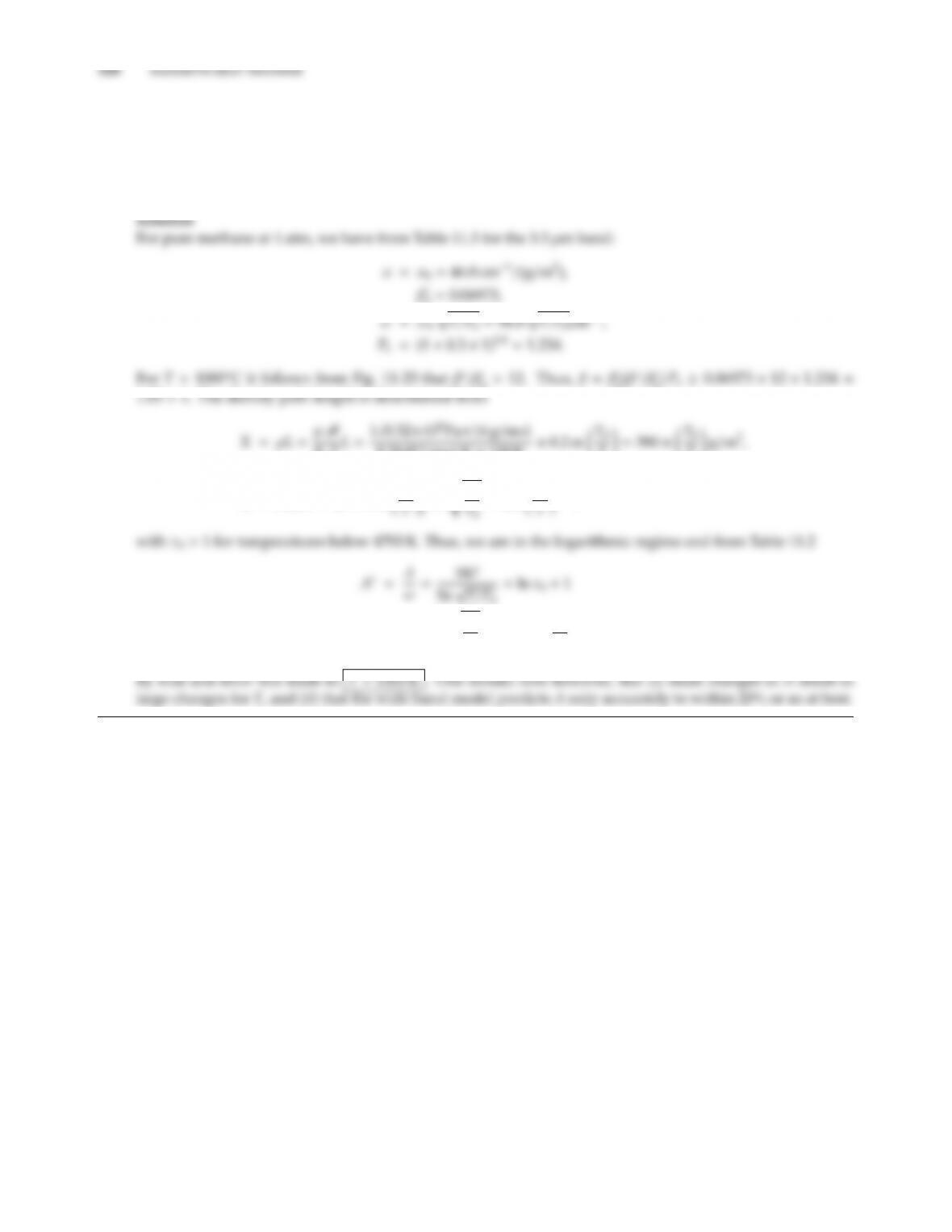11.35 One method of measuring the temperature of a high-temperature gas is to determine the total band absorp-
tance of a vibration-rotation band of the gas under the prevailing conditions. Consider a 20 cm thick layer of
pure methane, CH4, at 1 atm pressure. If the total band absorptance of the 3.3µm band is 587 cm1, what is
the temperature of the CH4?
Note: Such instruments are generally used only for T>1000 C.
0=0.06973,
0>12. Thus, β=β
0(β
0)Pe0.06973 ×12 ×1.234 =
RuTL=1.0132×105Pa×16 g/mol
8.3145 J/mol K ×100 K ×0.2 m T0
T=390 ×T0
Tg/m2,
and
τ0=αX=46 ×390 T0
=320 T0
,
10.482 rT0
T=ln "320 T0
T3/2#+1.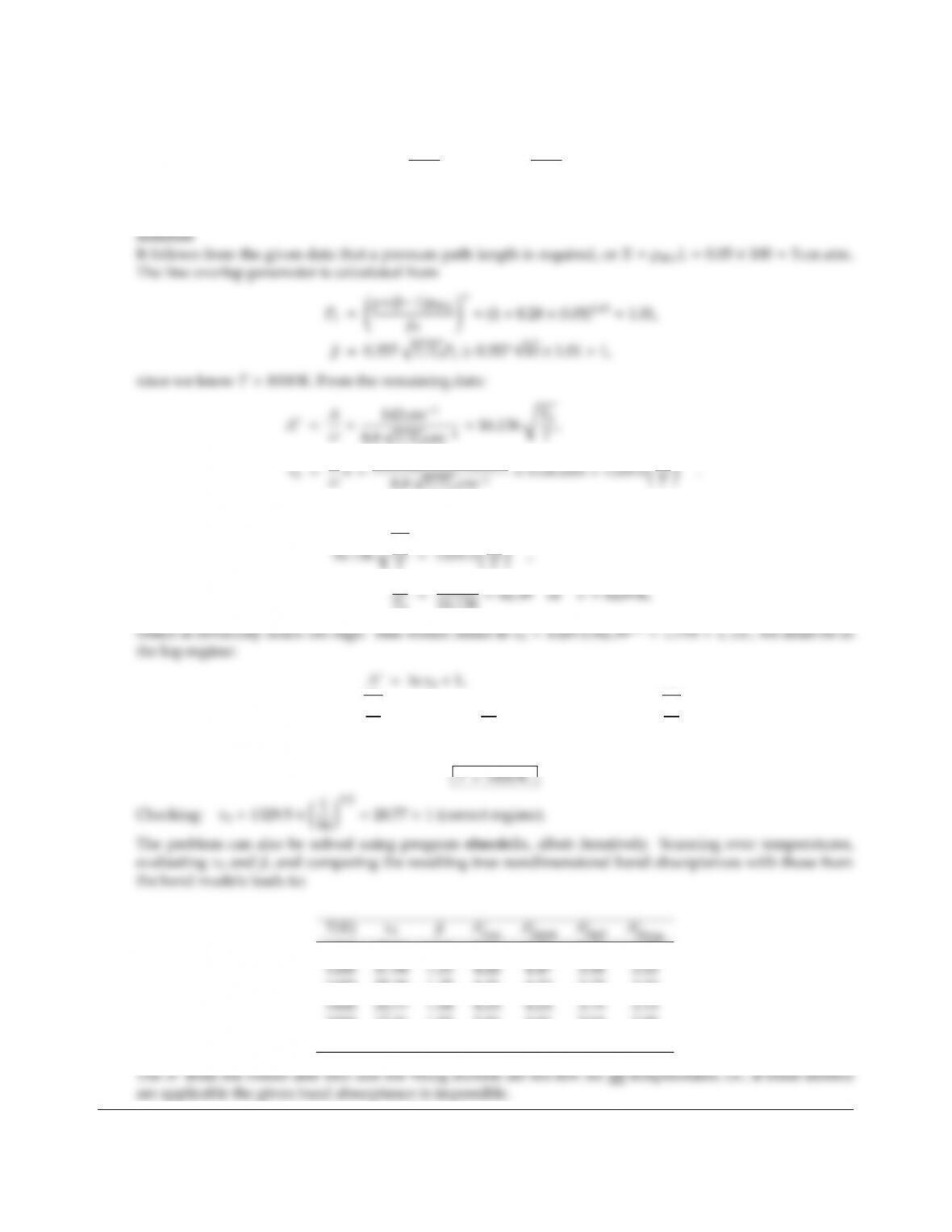CHAPTER 11 329
11.37 A mixture of nitrogen and sulfur dioxide (with 5% SO2by volume) is at 1 atm total pressure. To measure
the temperature of the mixture in a furnace environment (T>1000 K), an instrument is used that measures
total band absorptance for the strong SO2-band centered at ηc=1361 cm1. For that band it is known that
α=2340 (T0/T) cm2atm1,β=0.357 T/T0Pe,ω=8.8T/T0cm1,b=1.28 and n=0.65. What is the
temperature of the mixture if the total band absorptance has been measured as 142 cm1for a 1 m thick gas
layer?
If we were in the linear regime A=τ0, or
T=1329.5T0
T3/2
T
T0
16.136 =82.39 or T=8239 K,
16.136 rT0
T=ln "1329.5T0
T3/2#+1=8.193 +3 ln rT0
T
By trial and error this leads to
1000 42.04 1.14 5.10 4.74 3.82 3.77
1400 25.38 1.35 4.31 4.23 3.37 3.33
1800 17.41 1.53 3.86 3.86 3.04 3.00
2500 10.60 1.80 3.23 3.36 2.61 2.56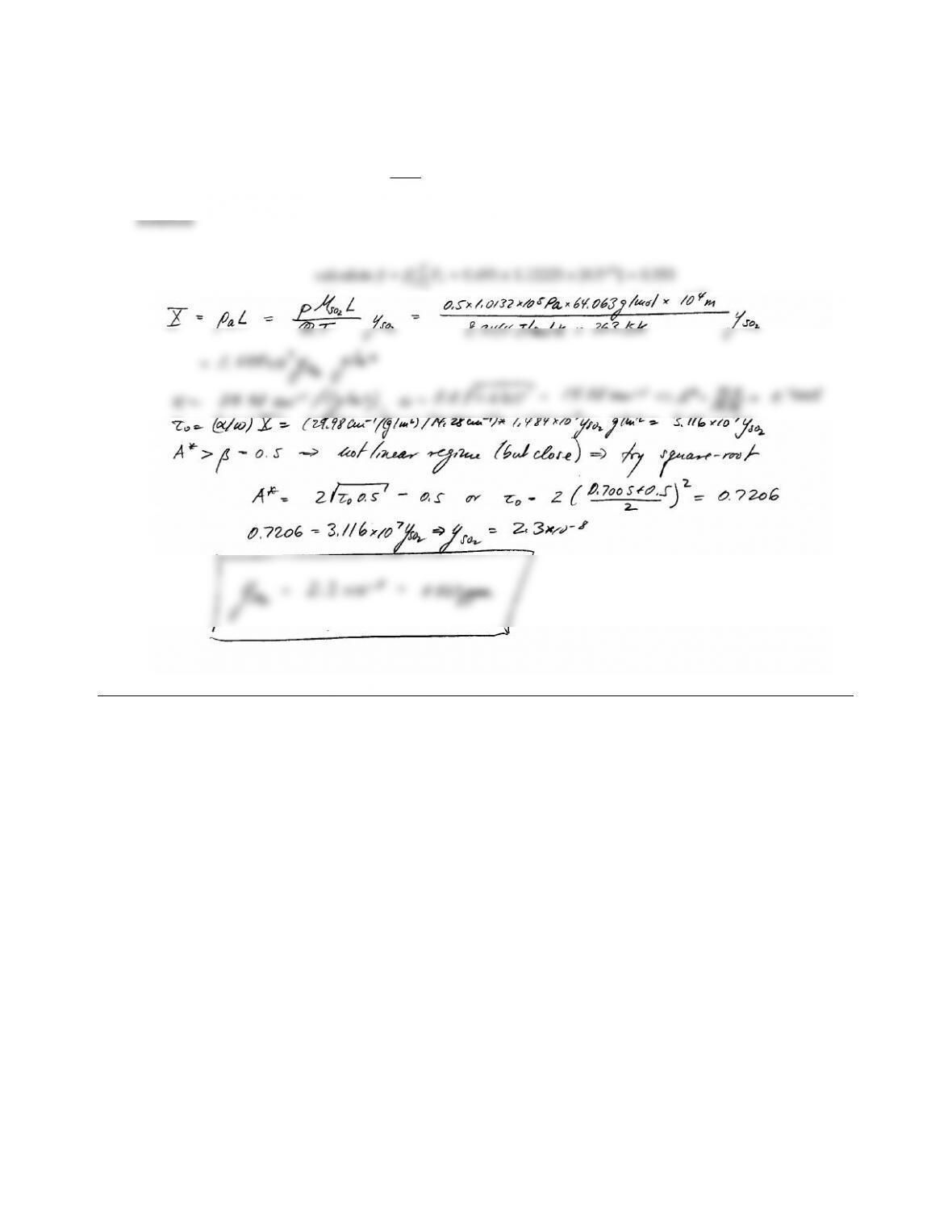11.38 The Earth’s pollution with sulfur dioxide (SO2)is determined by measuring the transmission of a light beam
from a satellite. Assuming that the band absorptance of the 7.3 µm band has been measured as 10.0 cm1, and
that the atmosphere may be approximated as a 10 km thick isothermal layer of nitrogen (with a trace of SO2)
at 0.5 atm and 10C, determine the volume fraction of SO2. Use wbmso2 from Appendix F to calculate the
overlap parameter βor use β0.357T/T0Pe.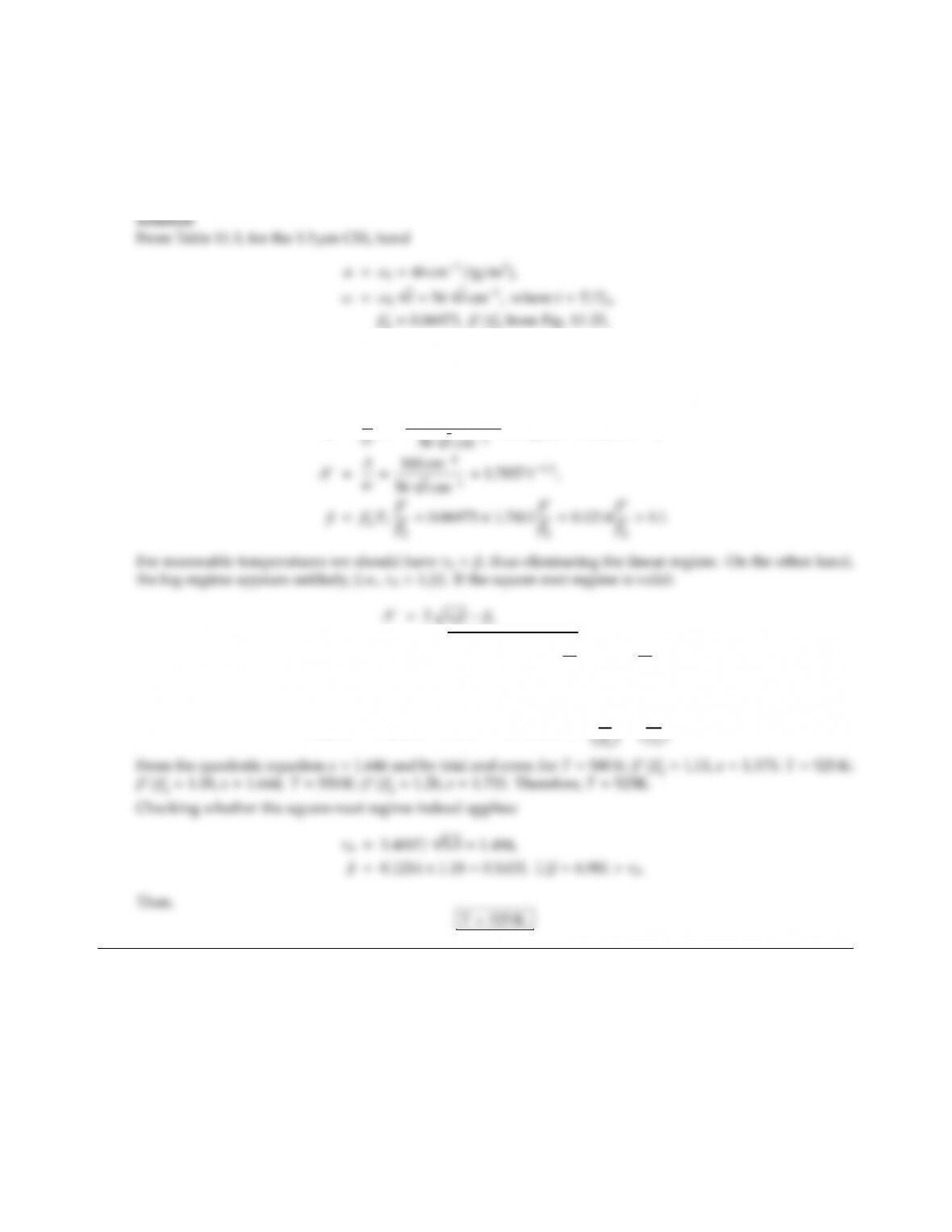CHAPTER 11 331
11.39 To determine the average atmospheric temperature on a distant planet, the total band absorptance for the
3.3µm CH4band has been measured as A3.3=100 cm1. It is known from other measurements that methane
is a trace element in the atmosphere (which contains mostly nitrogen and whose total pressure is 2 atm), and
that the absorption path length for methane on that planet, for which A3.3was measured, is 4.14 g/m2. What
is the temperature?
0=0.06973, β
0from Fig. 11-25,
n=0.8,b=1.3,Pe=h2+0.3pCH4)i0.820.8=1.7411.
Thus,
τ0=α
1.7857 t1/2=2s3.4007 t1/20.1214 β
β
00.1214 β
β
0
,
or
0.1214x21.2851x+1.7857 =0,where x= β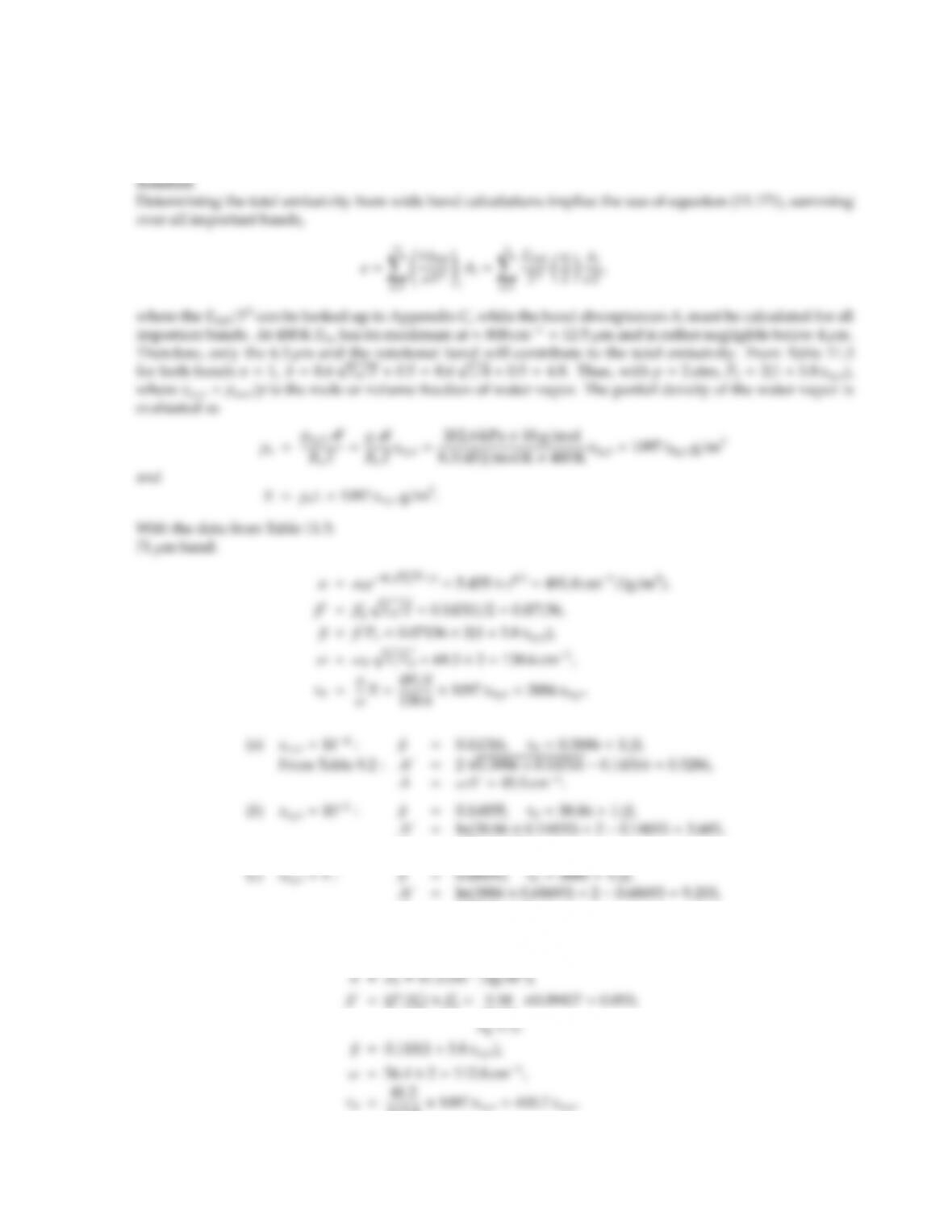11.40 Using the exponential wide band model, evaluate the total emissivity of a 1 m thick layer of a nitrogen–water
vapor mixture at 2 atm and 400 K if the water vapor content by volume is (a) 0.01%, (b) 1%, or (c) 100%.
Compare with Leckner’s model using subroutine totemiss.
A=499.6 cm1.
A=1275.5 cm1.
6.3µm band:
0)×β
0=0.58
|{z}
112.8×1097 xH2O=400.7xH2O.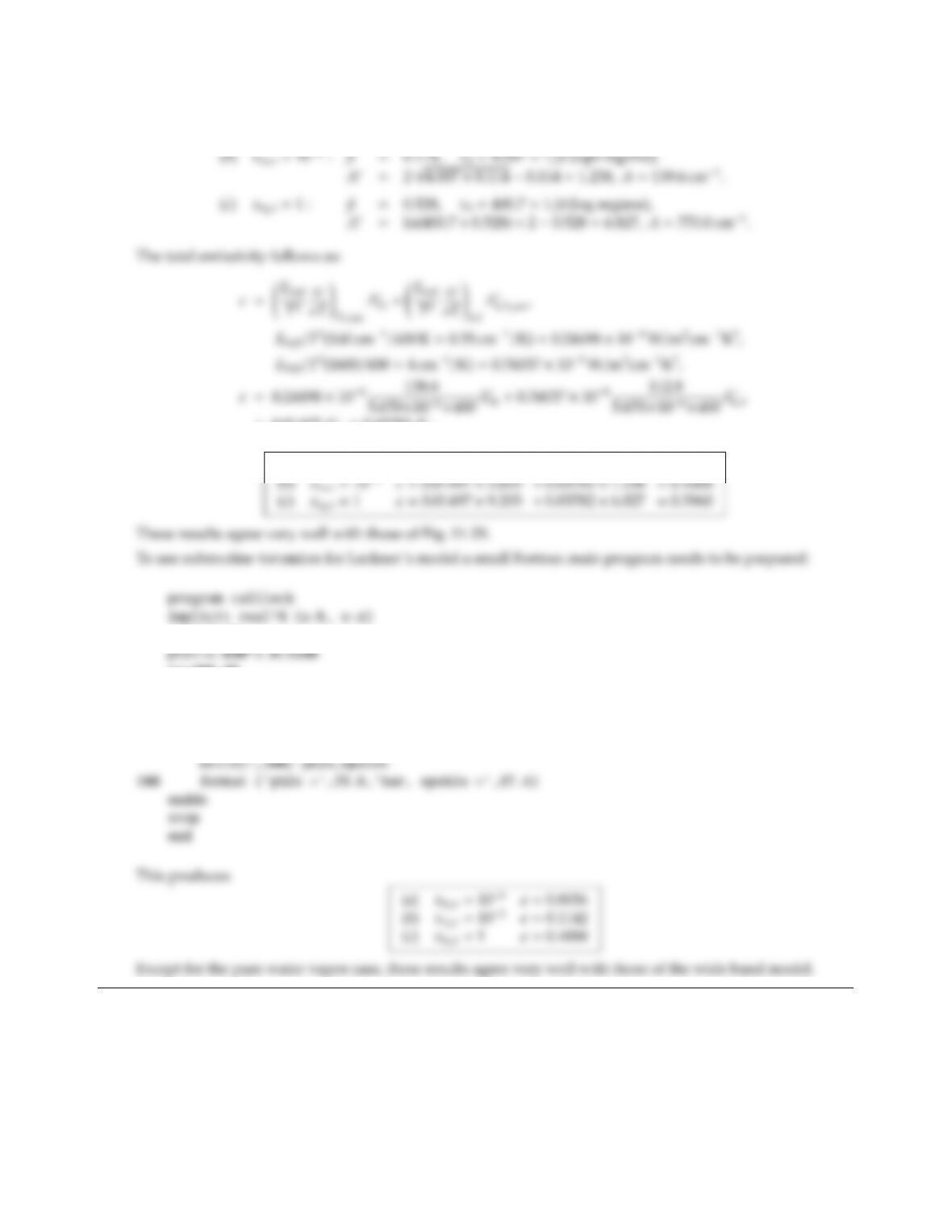CHAPTER 11 333
(a)xH2O=104:β=0.110, τ0=0.0401 < β (linear regime),
A=τ0,A=ωτ0=4.5 cm1.
=0.01497 A
71 +0.03782 A
6.3
(a)xH2O=104ǫ=0.01497 ×0.3286 +0.03782 ×0.0401 =0.0064
pco2=0.d0
tg=400.d0
xl=1.d2
do i=1,3
ph2o=1.d1**(2*i-6)*ptot
call totemiss (ph2o,pco2,ptot,tg,xl,epsh2o,epsco2,epstot)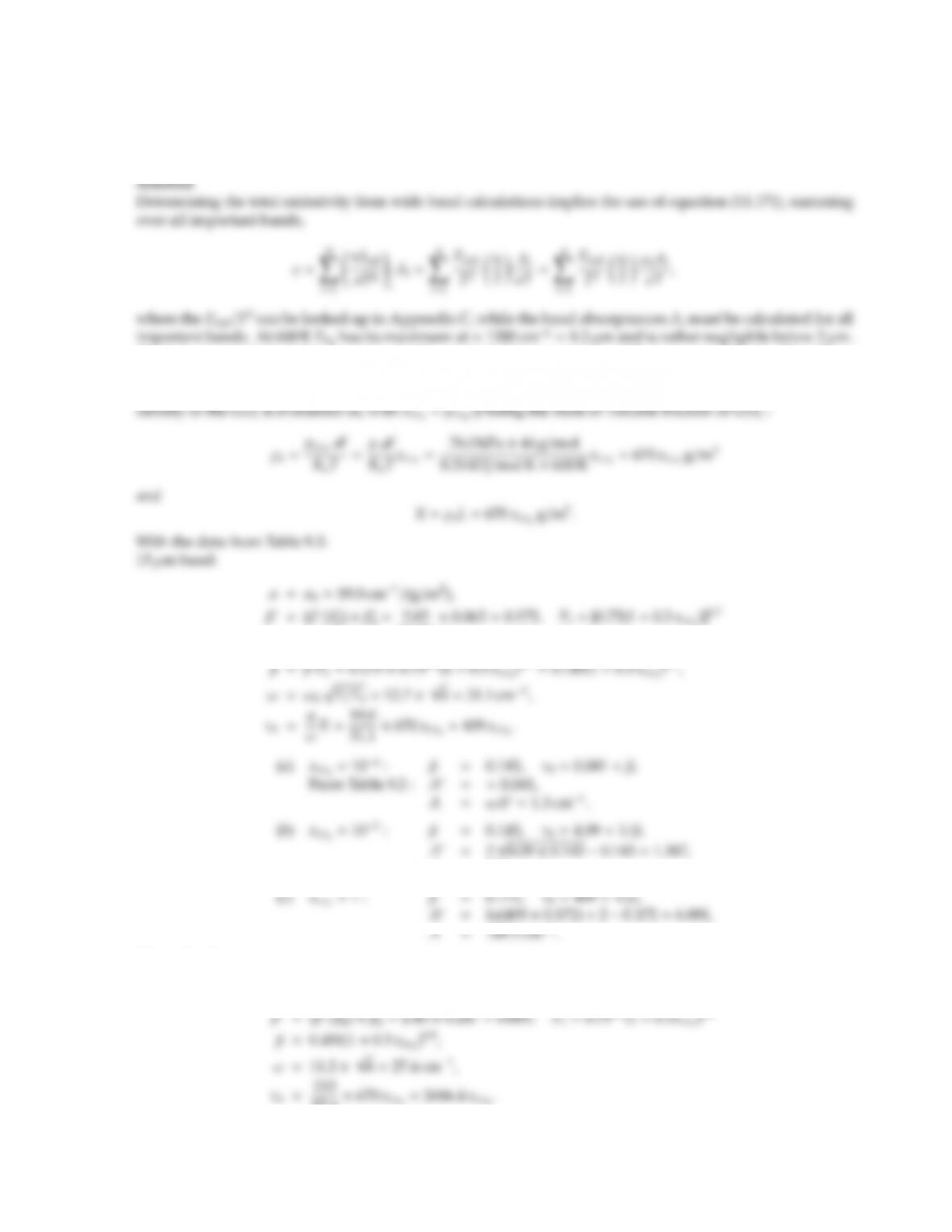11.41 Using the exponential wide band model, evaluate the total emissivity of a 1 m thick layer of a nitrogen–CO2
mixture at 0.75 atm and 600 K if the CO2content by volume is (a) 0.01%, (b) 1%, or (c) 100%. Compare with
Leckner’s model using subroutine totemiss.
The 9.4 µm and 10.4 µm bands are negligibly weak (only important for CO2laser generation). The 2.7µm
band is relatively weak and, combined with its spectral position, is almost negligible, but will be included
here. Therefore, only the 2.7µm, 4.3µm and 15 µm bands will contribute to the total emissivity. The partial
0)×β
0=2.82
|{z}
Fig. 9-13
A=43.1 cm1.
4.3µm band:
α=α0=110.0 cm1/(g/m2),
27.4×670 xCO2=2686.4xCO2.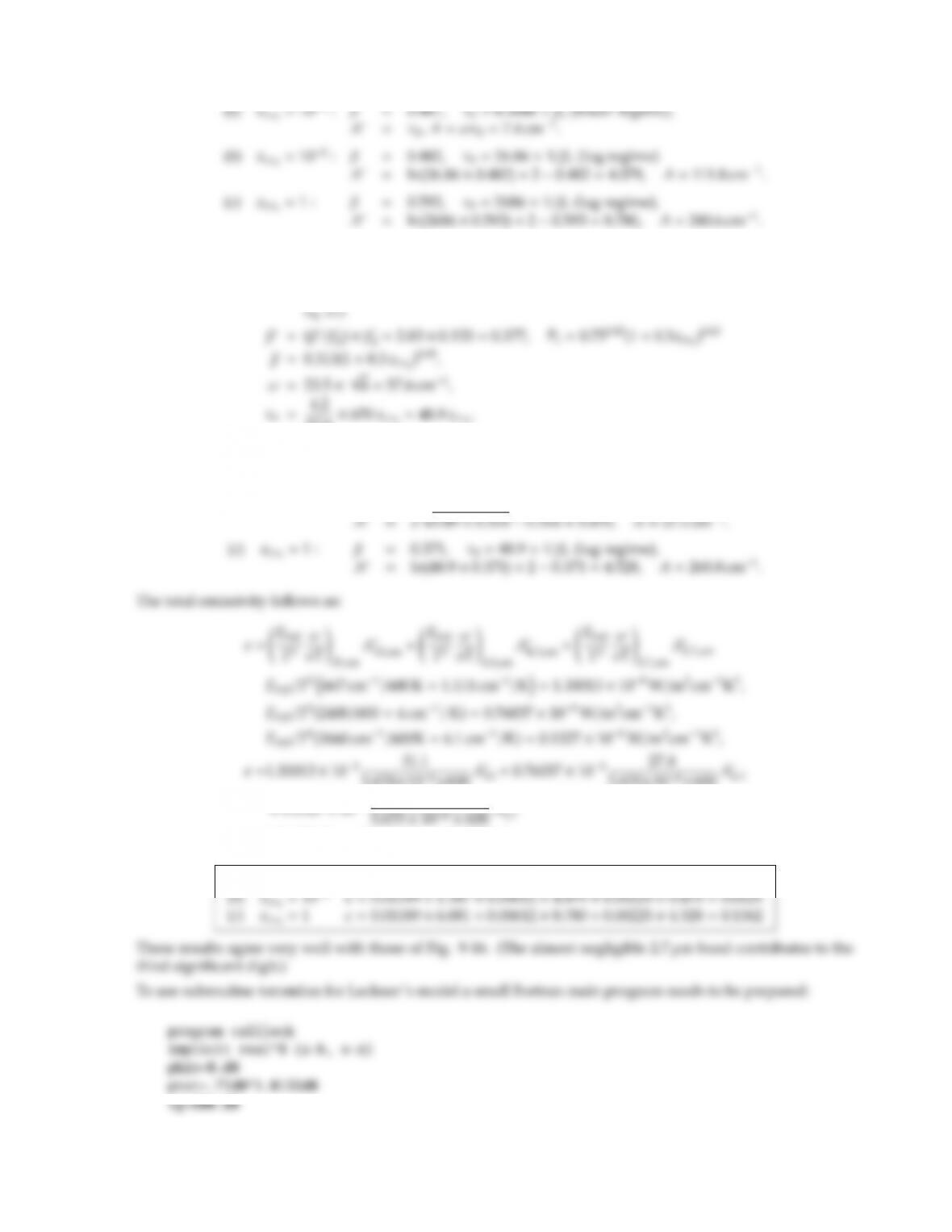CHAPTER 11 335
2.7 µm band:
α=(α/α0)
| {z }
α0=1.044 ×4.0=4.2 cm1/(g/m2),
57.6×670 xCO2=48.9xCO2.
(a)xCO2=104:β=0.313, τ0=5×103< β, (linear regime),
A=τ0,A=0.29 cm1.
(b)xCO2=102:β=0.314, τ0=0.49 <1/β, ( sqrt regime)
5.670×108×600 A
5.670×108×600 A
+0.1327 ×10857.6
5.670 ×108×600 A
=0.01189 A
15 +0.0612 A
4.3+0.00225 A
2.7
(a)xCO2=104ǫ=0.01189 ×0.041 +0.00612 ×0.269 +0.00225 ×0.005 =0.0021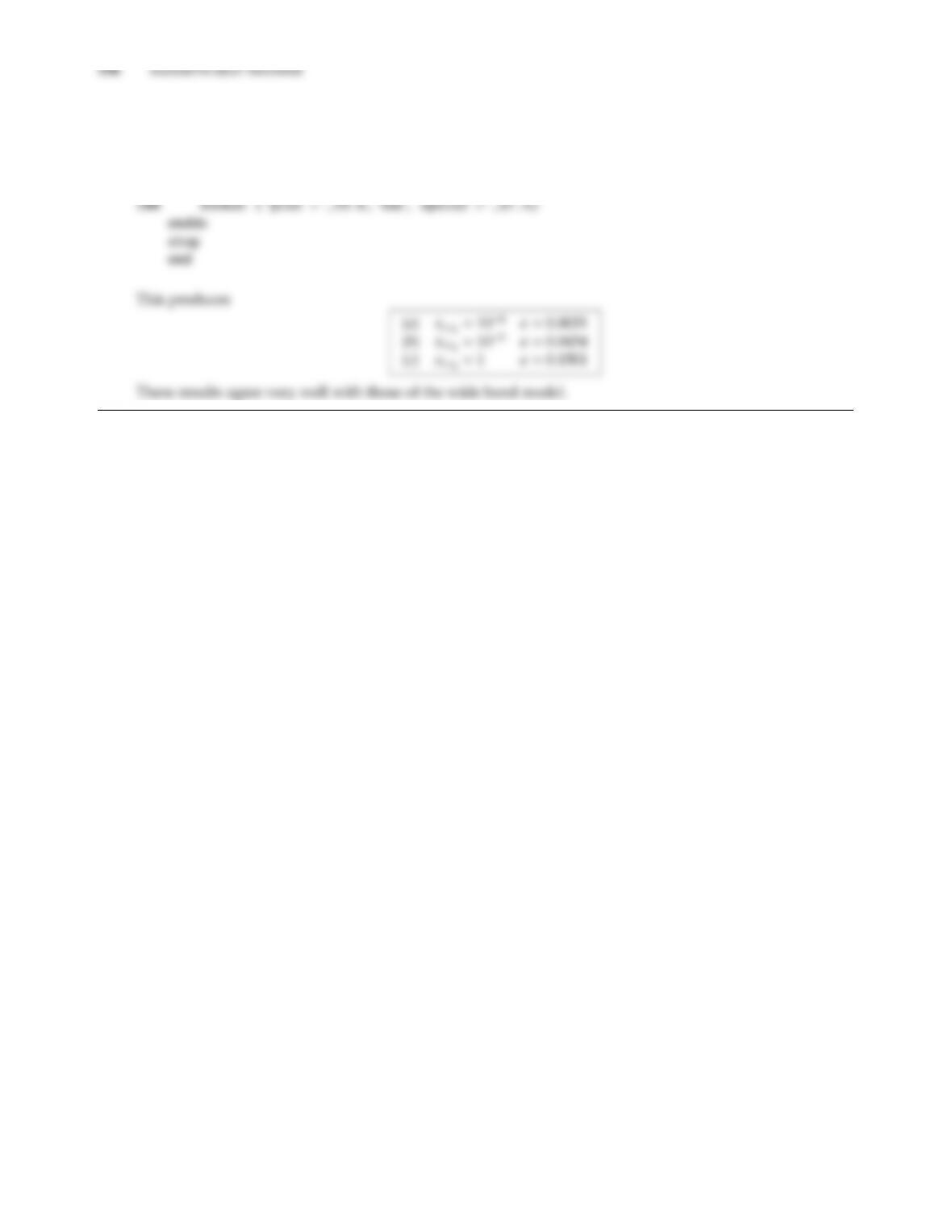xl=1.d2
do i=1,3
pco2=1.d1**(2*i-6)*ptot
call totemiss (ph2o,pco2,ptot,tg,xl,epsh2o,epsco2,epstot)
write(*,100) pco2,epstot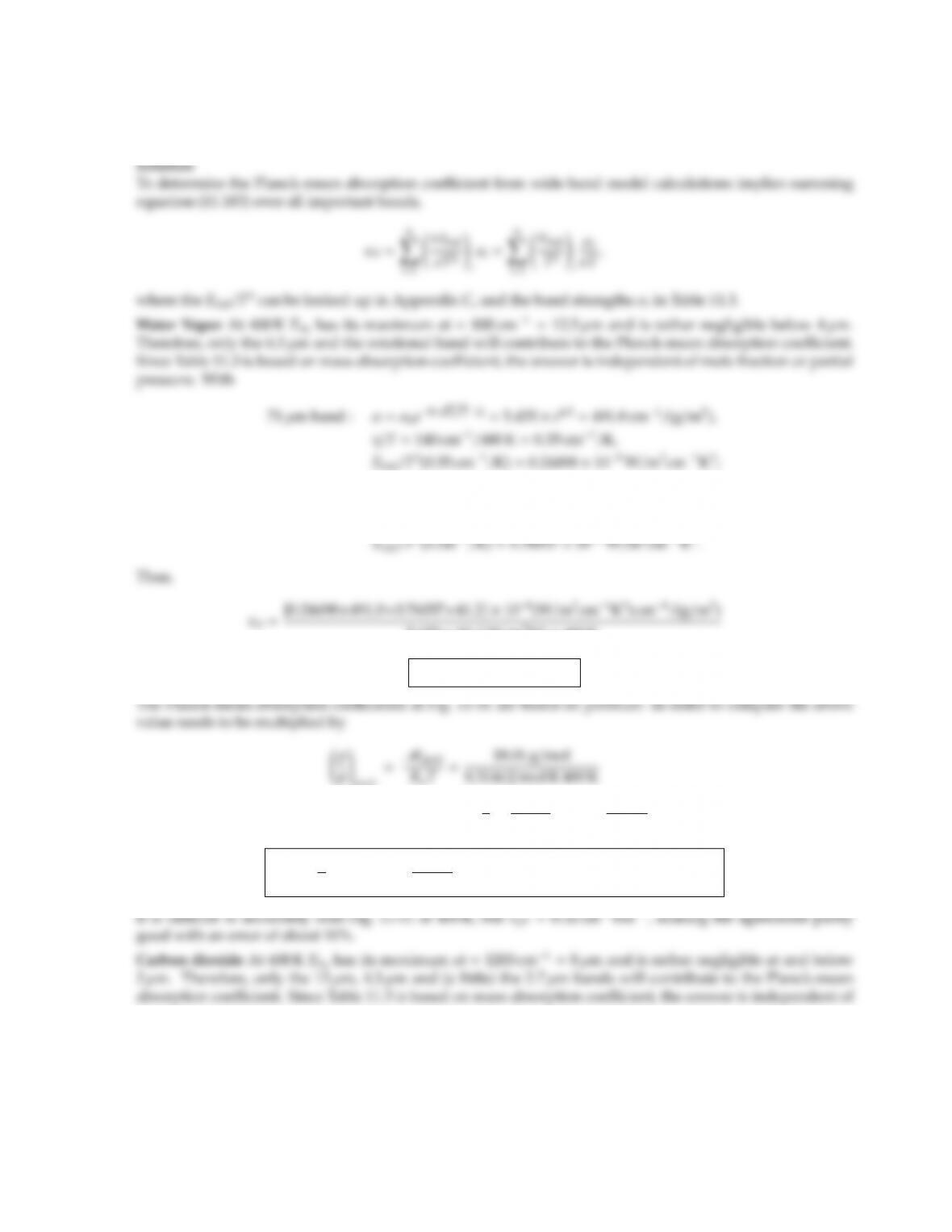CHAPTER 11 337
11.42 Evaluate the Planck-mean absorption coecients for the two gases in Problems 11.40 and 11.41, based on the
data given in Table 11.3. Compare the results with Fig. 11-31.
6.3µm band : α=α0=41.2 cm1/(g/m2),
η/T=1600 cm1/400 K =4 cm1/K,
5.670 ×108W/m2K4×400 K
κP=6.7×102(g/m2)1.
=5.415 ×103g
J×105Pa
1 bar =541.5g
m3bar,
κpP = ρ
p!κρP=541.5g
m3bar ×6.7×102(g/m2)1=0.363 cm1bar1.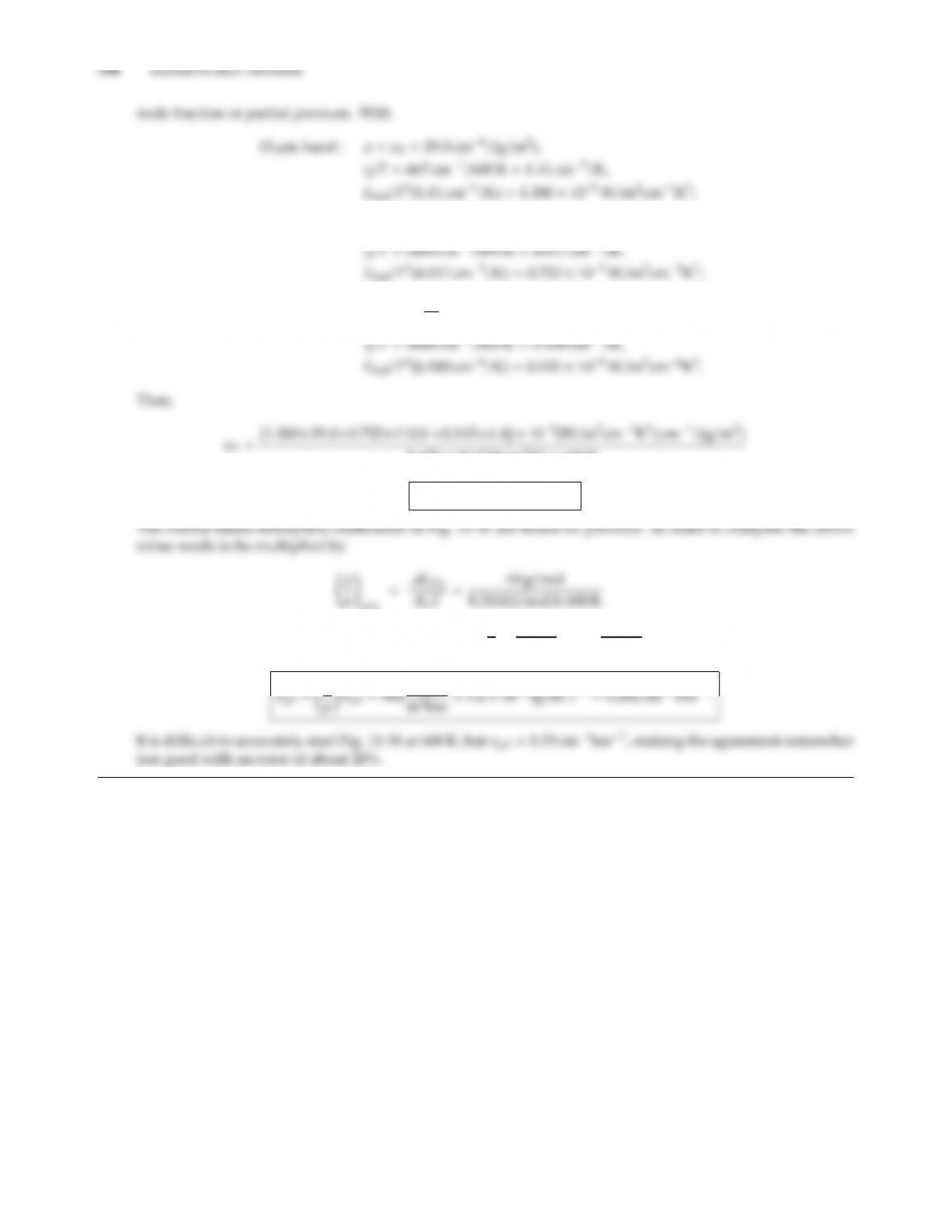4.3µm band : α=α0=110.0 cm1/(g/m2),
2.7µm band : α=α0×α
α0
=4.0 cm1/(g/m2)×1.1=4.4 cm1/(g/m2),
5.670 ×108W/m2K4×600 K
κP=3.2×102(g/m2)1.
=8.820 ×103g
J×105Pa
1 bar =882 g
m3bar,

## Trusted by Thousands ofStudents

Here are what students say about us.